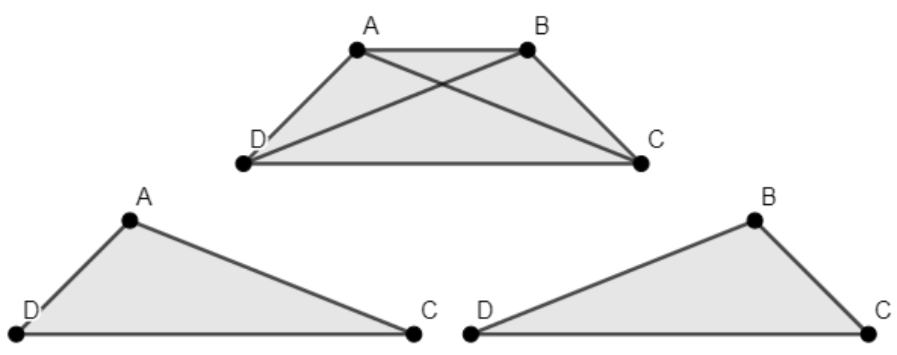Courses
Courses for Kids
Free study material
Free LIVE classes
MoreLIVE
Join Vedantu’s FREE Mastercalss

# Prove that the base angles of an isosceles trapezium are equalVerified
268.5k+ views
Hint: A trapezium is a cyclic quadrilateral with one pair of parallel sides. An isosceles trapezoid has one pair of parallel sides and another pair of congruent sides (means equal in length). The diagonals are of equal length in isosceles trapezoid and by SSS congruency we will prove that base angles are equal.

Complete step-by-step solution:Draw an isosceles trapezium ABCD.
Join the diagonal BD and AC in the isosceles trapezium ABCD.
By drawing the diagonals inside the trapezium we can see triangles $\Delta ADC$and $\Delta BCD$ are formed.
We know we can prove equal any angles or sides of two triangles if they are congruent.
Congruency theorem is the method used to prove sides or angles equal in the case of triangles.
In $\Delta ADC$and $\Delta BCD$
We know opposite sides are equal in an Isosceles trapezium.
We know the diagonals are equal in an Isosceles trapezium.
AC = BD
We know the side DC is common in both the triangles implies,
DC = DC
So, we can say that by (side-side-side) SSS congruency the two triangles are congruent.
$\Delta ADC\cong \Delta BCD$ (SSS postulate)
$\angle D=\angle C$ (Congruency property)
Hence proved that base angles are equal.

Note: In the above problem we proved that two triangles are similar by SSS postulate. SSS postulate states that, if the three sides of one triangle are equal to three sides of another triangle, then we say that two triangles are congruent.

Last updated date: 21st Sep 2023
Total views: 268.5k
Views today: 2.68k